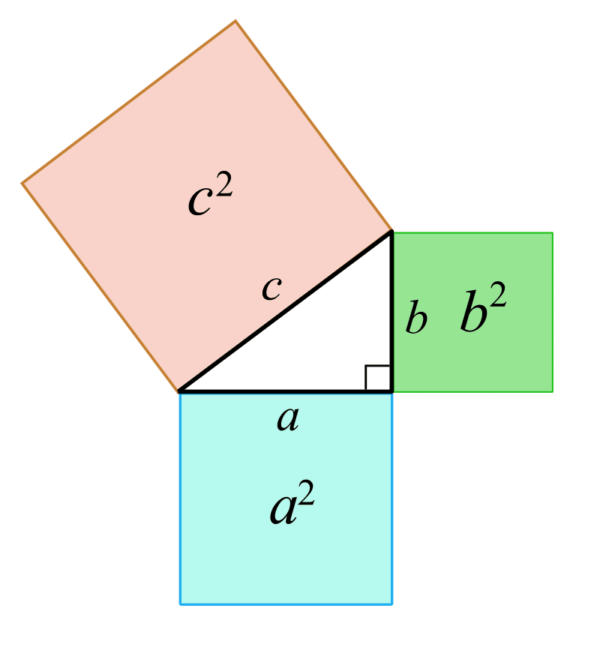# Pythagorean Theorem

Last updated on by ​Arikaran KumarImage courtesy: wikimedia.org

In the, we learned about triangles and their properties as well as how to locate a triangle's area and a missing angle. This blog teaches us how to locate the triangle's missing side.

The Pythagorean Theorem, created by Pythagoras of Samos, can be used to solve for the missing sides of a right triangle. It states that the sum of the squares of the legs of the triangle is equal to the square of the hypotenuse.Figure #1Figure #2

The theorem can be written asWhereandrepresent either of the legs, and, the hypotenuse.

Using this theorem, the solution for each side can also be solved asNow that we have talked a bit about what the Pythagorean Theorem can be used for, let us examine at one of the many ways we can derive this formula.

## Pythagorean Theorem ProofFigure #3

Look at Figure #3. We have a small green square inscribed in a larger beige square. The small green square has side lengths. Since the green square is inscribed in the beige square, it creates 4 identical right triangles with the legs measuringand, and a hypotenuse measuring. Using this information, we can start creating equations to solve for each side of the right triangles.

Let’s first find the area of the beige square.

The area of a square isThe length of the square is equal to.

The width, since all sides of a square are equal, is also. So,Distributing, we getThe area of the green square isNext, let’s get the areas of the triangles. The area of a triangle isFinding the area of one of the triangles, we can see that the base isand the height is.So, the area can be represented asSince all 4 triangles are identical, we can obtain the total area of all four triangles by multiplying the area of 1 triangle by 4.Using this new information, we can form another equation for the total area of the beige square. We can add the areas of the 4 right triangles to the area of the green square to get the total area of the beige square.We now have two equations for the area of the beige square. We haveandNow we can set both equations equal to each other and solve forSubtractingfrom both sides we getSimplifying, we getNow that we are done proving the Pythagorean Theorem, let’s apply it to some examples.

## Examples

Find the missing side,, of each given triangle

#1Figure #4

Remember:

The Pythagorean Theorem states,Whereandare the legs andis the hypotenuse.

Using the theorem, we can create the following equation for the triangle in Figure #4:Simplifying, we getIsolatingwe get#2Figure #5

In Figure #5, we are missing the value of one of the legs of the triangle. To solve for, we can create the equation:Simplifying, we getIsolatingwe getAuthor: Mr. Vernon Sullivan, is a tutor at FPLA Miami, FL HQ premier 1-on-1 tutoring center. He teaches Algebra, Geometry, Pre-Cal, ACT, SAT, SSAT, HSPT, PERT, ASVAB and other test prep programs.

Mrs. Emimmal Sekar and Mr. Arikaran Kumar Proofread this article. Mr. Arikaran Kumar manages the website and the social media outreach.

FPLA Action Plan for COVID-19 Crisis
FPLA Action Plan for COVID-19 CrisisImage courtesy CDC, AtlantaHealth and Well-being are Important to Us.We are carefully monitoring and following[...]